1-D Kinematics - Lesson 2 - Describing Motion with Diagrams

# Ticker Tape DiagramsImagine a device that could identify the position of a moving object at constant intervals of time - for instance, every second or every 1/10-th second or even every 1/60-th second. Perhaps such a device could track the location of a object moving in 1-dimension by placing a dot on a strip of paper. The trail of dots would represent the motion of the object as it changes its position over the course of time.

Believe it or not, there is such a device - it's called a ticker tape timer. Before the advent of  computers in Physics labs, a common way of analyzing the motion of objects in physics labs was to perform a ticker tape analysis. A long tape was attached to a moving object and threaded through a device that placed a tick upon the tape at regular intervals of time - say every 0.10 second. As the object moved, it dragged the tape through the "ticker," thus leaving a trail of dots. The trail of dots provided a history of the object's motion and therefore a representation of the object's motion.### Dot Diagrams

While the use of ticker tape analyses in Physics labs has mostly been replaced by the use of computer-interfaced motion detectors, the use of ticker tapes or motion diagrams still persists in our Physics curriculum due to the visual nature of representing an object's motion. Such diagrams are referred to as dot diagrams, motion diagrams, oil drop diagrams, and (still) ticker tape diagrams.

The distance between dots on a dot diagram represents the object's position change during that time interval. A large distance between dots indicates that the object was moving fast during that time interval. A small distance between dots means the object was moving slow during that time interval. Dot diagrams for a fast- and slow-moving object are depicted below.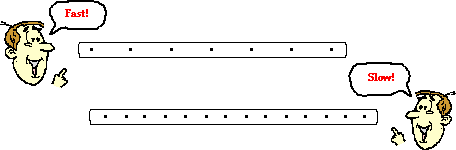The analysis of a dot diagram will also reveal if the object is moving with a constant velocity or accelerating. A changing distance between dots indicates a changing velocity and thus an acceleration. A constant distance between dots represents a constant velocity and therefore no acceleration. Dot diagrams for objects moving with a constant velocity and with an accelerated motion are shown below.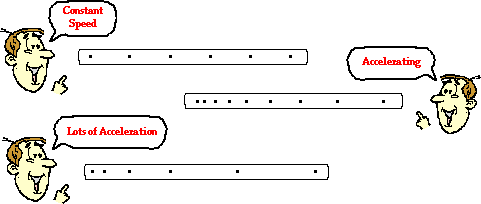And so dot diagrams provide one more means of representing various features of the motion of objects.

### We Would Like to Suggest ...Sometimes it isn't enough to just read about it. You have to interact with it! And that's exactly what you do when you use one of The Physics Classroom's Interactives. We would like to suggest that you combine the reading of this page with the use of our Name That Motion Interactive. It is found in the Physics Interactive section and allows a learner to apply concepts of speed, velocity, acceleration and ticker tape diagrams.

Visit Name That Motion.

Ticker tape diagrams are sometimes referred to as oil drop diagrams. Imagine a car with a leaky engine that drips oil at a regular rate. As the car travels through town, it would leave a trace of oil on the street. That trace would reveal information about the motion of the car. Renatta Oyle owns such a car and it leaves a signature of Renatta's motion wherever she goes. Analyze the three traces of Renatta's ventures as shown below. Assume Renatta is traveling from left to right. Describe Renatta's motion characteristics during each section of the diagram. Click the button to check your answers.

 1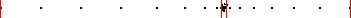2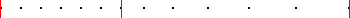3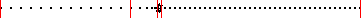Next Section: